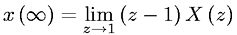Equations > Signal Processing > Z Transform Properties > Z-transform final value theorem. Valid only if polues of (z-1)X(z) are inside the unit circle.

### Z-transform final value theorem. Valid only if polues of (z-1)X(z) are inside the unit circle.Latex Code:

MathML Code:

 $x\left(\infty \right)=\underset{z\to 1}{lim}\left(z-1\right)X\left(z\right)$

MathType 5.0: